# Conditional Statements in C++: if , if..else, if-else-if and switch statements

11 Sep 2023
Beginner
3.62K Views

## Conditional Statements in C++: An Overview

The flow of a program can be managed using conditional statements in C++. If a condition is true, the "if" statement tests it and then executes the code. When the condition is false, "else" can be used to specify an alternate course of action. You can check several criteria sequentially using the "else if" statement. For multi-way branching based on the result of an expression, use the "switch" statement. These statements, which control program flow based on logical circumstances, are crucial for developing adaptable and responsive C++ programs.

## What are Conditional Statements in C++ programming

In C++, conditional statements are control structures that let code make decisions. The "if" clause tests a condition and runs code if it is true. "Else" can be used to denote a different course of action. While "switch" allows for multi-case selection, "else if" supports numerous circumstances. They regulate the flow of the program using logical tests.

## Types of Conditional Statements in C++ language

Following are the types of conditional statements in C++:

• if Statement
• if-else Statement
• Nested if Statement

### If Statement in C++

If statements in C++ are one of the most simple statements for making a decision in a program. It is used for deciding whether a certain block of the statement of a single statement will be executed or not. If the conditions are turned out to be true then it will execute the statements otherwise not.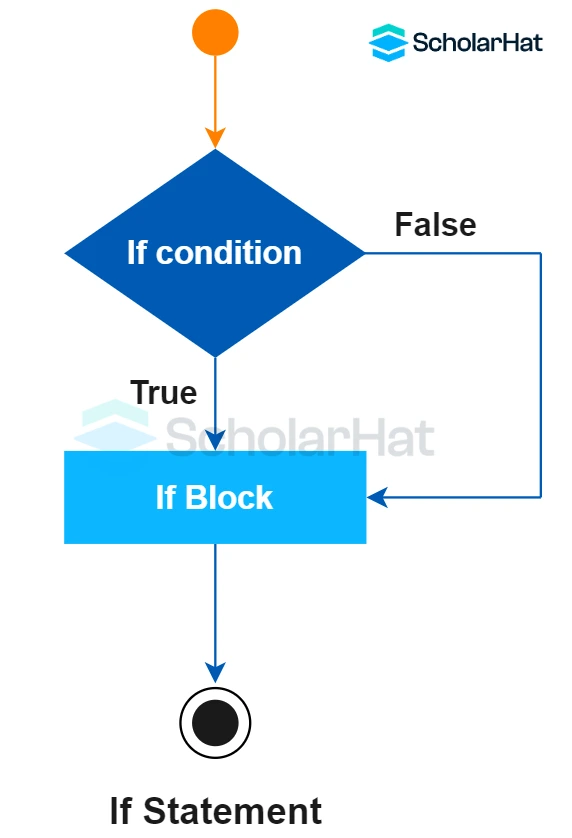#### Syntax

``````if(condition)
{
// Statements to execute if
// condition is true
}
``````

#### Example

``````// C++ program to understand If statement
#include <iostream>
using namespace std;
int main()
{
int i = 11;
if (i > 15)
{
cout << "11 is greater than 15";
}
cout << "I am Not in if";
}``````

This C++ code initializes the integer 'i' to 11 and then checks to see if it is greater than 15 using an "if" statement. It outputs "I am Not in if" to the console because it isn't.

#### Output

``````I am Not in if
``````

### If-else Statement in C++

If the value of If statements returned to be a false statement then it needs an else statement to execute the program. The programmers can use else statements with If statements for executing a single code of statement or any block of code of the statements when the condition is false.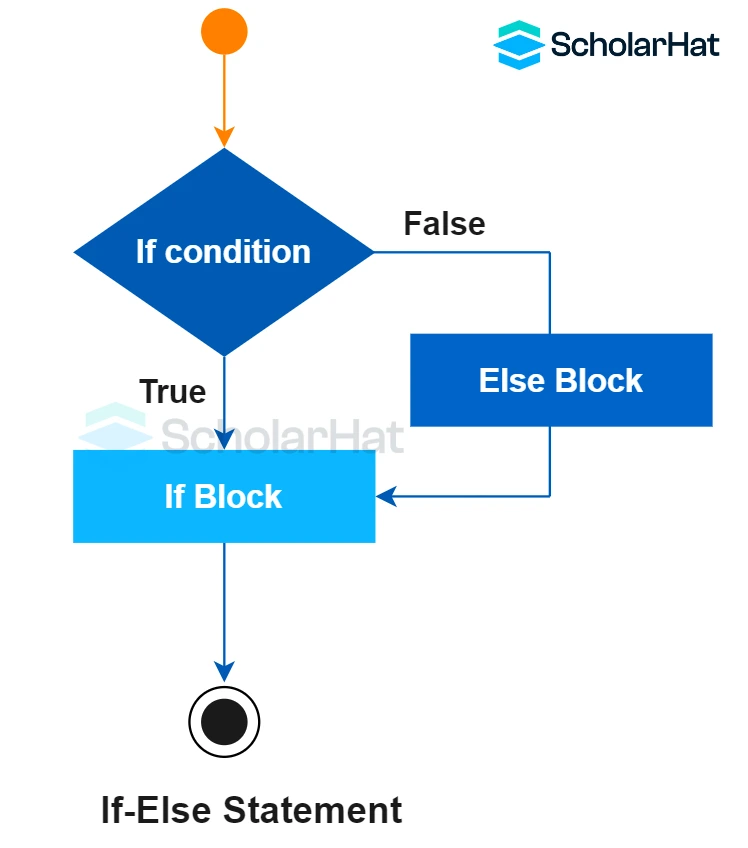#### Syntax

``````if (condition)
{
// Executes this block if
// condition is true
}
else
{
// Executes this block if
// condition is false
}
``````

#### Example

``````// C++ program to understand the if-else statement
#include <iostream>
using namespace std;
int main()
{
int A = 16;
if (A < 15)
cout << "A is smaller than 15";
else
cout << "A is greater than 15";
return 0;
}
``````

This C++ programme initializes the integer 'A' to 16 and then checks to see if it is less than 15 using a "if-else" statement. It outputs "A is greater than 15" to the console because it isn't.

#### Output

``````A is greater than 15
``````

### Nested If-else Statement in C++

A nested if else statement in C++ programming language is mainly targeted at another if statement which means an if statement inside another if statement. This is how nested if else statements execute any block of codes in the C++ programming language.

#### Syntax

``````if (condition1)
{
// Executes when condition1 is true
if (condition2)
{
// Executes when condition2 is true
}
}
``````

#### Example

``````// C++ program to understand nested-if statement
#include <iostream>
using namespace std;
int main()
{
int A = 10;
if (A == 10)
{
// First if statement
if (A < 15)
cout << "A is smaller than 15\n";
// Nested - if statement
// Will only be executed if
// statement above is true
if (A < 12)
cout << A is smaller than 12 too\n";
else
cout << "A is greater than 15";
}
return 0;
}``````

To check conditions, this piece of C++ code uses nested "if" statements. It first determines whether "A" is equal to 10, and then, within that block, it determines whether "A" is less than 15 and less than 12. When both requirements are satisfied, it prints "A is smaller than 12 too." If not, it displays "A is greater than 15."

#### Output

``````A is smaller than 15
A is smaller than 12 too
``````

### If else if ladder Statement in C++

This if else if ladder statements in C++ give the user multiple options to execute the program. If the program is executed in a top-down format and one of the conditions control is turned out to be true then it is associated with the if statement. Then the other rest of the program will use the else if ladder to execute the program. After that, if none of the conditions are turned out to be true then it will execute the final else statement of the code block in the C++ programming language.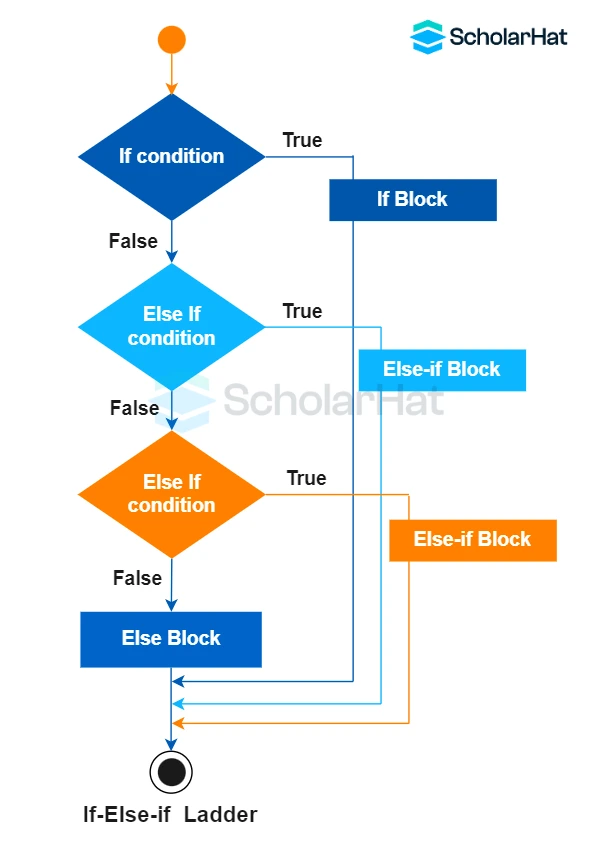#### Syntax

``````if (condition)
statement;
else if (condition)
statement;
.
.
else
statement;
``````

#### Example

``````// C++ program to understand if-else-if ladder
#include <iostream>
using namespace std;
int main()
{
int A = 20;
if (A == 10)
cout << "A is 10";
else if (A == 15)
cout << "A is 15";
else if (A == 20)
cout << "A is 20";
else
cout << "A is not present";
}``````

This "if-else-if" ladder is shown in this section of C++ code. It sequentially compares the value of 'A' to a number of conditions. It outputs "A is 20" as the matched condition & skips the remaining checks because "A" is 20.

#### Output

``````A is 20
``````

## Switch statements in C ++?

Switch statements in a programming language are a particular type of control mechanism that is used to allow the value of an expression or a variable to change the flow of the program execution by searching and mapping. Switch statements in C ++ help to execute one statement in multiple conditions.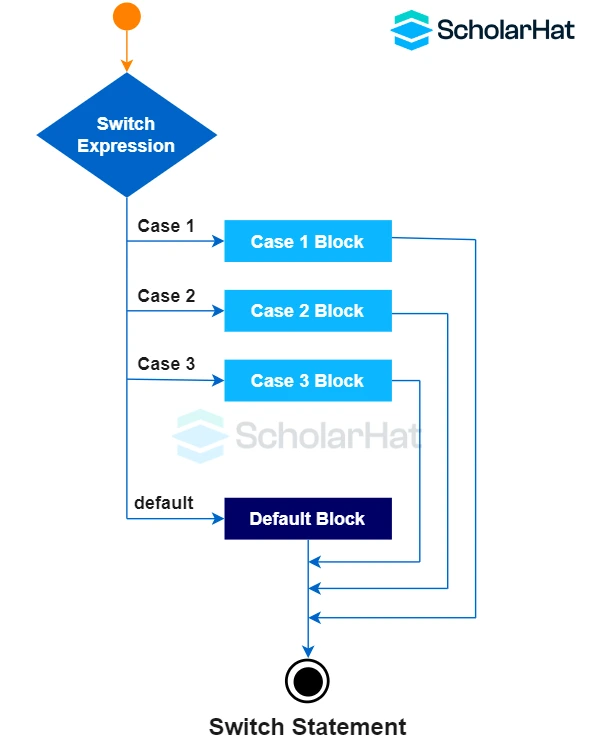#### Syntax

``````switch(expression)
{
case value1:
//code to be executed;
break;
case value2:
//code to be executed;
break;
......
default:
//code to be executed if all cases are not matched;
break;
}
``````

#### Example

``````#include <iostream>
using class std;
int main ()
{
int num;
cout<<"Enter a number to check grade:";
cin>>num;
switch (num)
{
case 20: cout<<"It is 20"; break;
case 30: cout<<"It is 30"; break;
case 40: cout<<"It is 40"; break;
default: cout<<"Not 20, 30 or 40"; break;
}
}
``````

This "if-else-if" ladder is shown in this section of C++ code. It sequentially compares the value of 'A' to a number of conditions. It outputs "A is 20" as the matched condition & skips the remaining checks because "A" is 20.

#### Output 1

``````Enter a number:
20
It is 20
``````

#### Output 2

``````Enter a number:
68
Not 20, 30 or 40
``````

## Conditional Operator in C++

Simple conditional statements can be implemented using the C++ conditional operator, also referred to as the ternary operator. It accepts a condition and evaluates and returns one of the two provided expressions based on whether the condition is true or false. The use of this operator enables programmers to quickly decide inside a single line of code.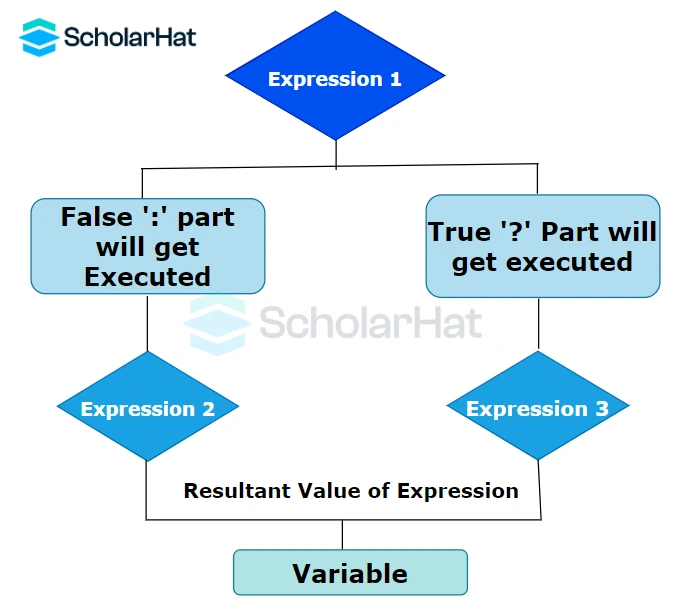#### Syntax

``````condition ? expression_if_true : expression_if_false;
``````

#### Example

``````#include <iostream>
``````using namespace std;
``````int main() {
`````` int num1 = 10;
`````` int num2 = 20;
`````` int max = (num1 > num2) ? num1 : num2;
`````` cout << "The maximum number is: " << max << endl;
`````` return 0;
``````}
``````

The conditional operator is used to determine which of two initialized numbers, "num1" and "num2," is greater. It prints to the console the maximum number ('num2', 20).

#### Output

``The maximum number is: 20``

## FAQs

### 1. What are the conditional statements in C++?

In C++, conditional statements are expressions like "if," "else," and "switch" that regulate program flow according to specific conditions.

### 2. What are the different types of statements in C++?

Expression statements, compound statements, selection statements (also known as conditional statements), and iteration statements (also known as loops) are the four primary types of statements in C++.

### 3. What are if-else and switch statements in C++?Conditional statements include "if-else" and "switch" in C++. While "switch" enables multi-case selection based on an expression's value, "if-else" gives branching based on true or false guidelines.

4. How do you write if statements in C++?

In C++, you use the syntax if (condition) /* code to execute if the condition is true */ to create an "if" statement.

5. What is the main difference between if-else and switch?

When comparing "if-else" to "switch," the key distinction is that "if-else" can handle complex conditions and expressions, whilst "switch" is usually used for basic value-based selection.

### 6. What is the difference between the if else and nested statements?

The main distinction between "if-else" and "nested if" is that in "if-else," if a single condition is satisfied, the corresponding block of code runs and then is terminated, whereas in "nested if," several conditions may be verified sequentially, and multiple blocks may run if multiple conditions are satisfied.

##### Summary

This article gives a vast idea about conditional statements in C++ language, including its various types with syntax and If-Else and Switch Statements in C++ with examples, which can be helpful for your understanding of C++ Language. If you've understood everything we went over in this blog post, congratulations! Now you can check out for a C++ certification. C++ if-else and switch statements provide you with different options to control the flow of a program based on user input or other conditions. If-Else Statements are executed from top to bottom, one after the other. Switch Statements test for a particular value and execute the corresponding case. You're now ready to start using if-else statements like a pro. Just remember the basic format and key points we covered, and you'll be writing if-else statements in your sleep in no time.

Similar Articles